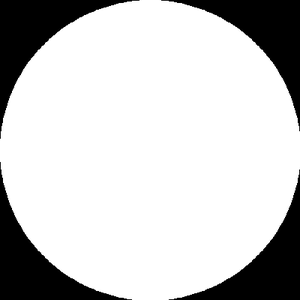Open in App
Not now

# Cropping an Image in a circular way using Python

• Difficulty Level : Expert
• Last Updated : 24 Feb, 2021

In this article, we will learn to crop an image circularly using a pillow library. Cropping an image circularly means selecting a circular region inside an image and removing everything outside the circle.

Approach:

• If you have an L mode image, the image becomes grayscale. So we create a new image with mode “L”.
• An image is created with a white circle in the middle with dimensions same as the input image.
• Convert a new image to an array.
• Convert original image from an array.
• Stack these two arrays together to crop out only the circular middle part.

Let’s take this initial image :Step 1: Import the module and read the image.

## Python3

 `import` `numpy as np``from` `PIL ``import` `Image, ImageDraw`` ` ` ` `img ``=` `Image.``open``(``"/content/gfg.jpeg"``)``display(img)`

Output:Step 2: Create an image.

We will use pieslice() function to get the circular part of the image in white, then we will superimpose the original image and the luminous image.

ImageDraw.Draw.pieslice() Same as arc, but also draws straight lines between the endpoints and the center of the bounding box.

Syntax: PIL.ImageDraw.Draw.pieslice(xy, start, end, fill=None, outline=None)

Parameters:
xy – Four points to define the bounding box. Sequence of [(x0, y0), (x1, y1)] or [x0, y0, x1, y1].
start – Starting angle, in degrees. Angles are measured from 3 o’clock, increasing clockwise.
end – Ending angle, in degrees.
fill – Color to use for the fill.
outline – Color to use for the outline.

Returns: An Image object in pieslice shape.

Code:

## Python3

 `h,w ``=` `img.size`` ` `# creating luminous image``lum_img ``=` `Image.new(``'L'``,[h,w] ,``0``) ``draw ``=` `ImageDraw.Draw(lum_img)``draw.pieslice([(``0``,``0``),(h,w)],``0``,``360``,fill``=``255``)``img_arr ``=` `np.array(img)``lum_img_arr ``=` `np.array(lum_img)``display(Image.fromarray(lum_img_arr))`

Output:Step 3: Stack these two arrays together to crop out only the circular middle part.

## Python3

 `final_img_arr ``=` `np.dstack((img_arr, lum_img_arr))``display(Image.fromarray(final_img_arr))`

Output:Below is the full implementation:

## Python3

 `import` `numpy as np``from` `PIL ``import` `Image, ImageDraw`` ` `img``=``Image.``open``(``"img.jpg"``)``display(img)`` ` `height,width ``=` `img.size``lum_img ``=` `Image.new(``'L'``, [height,width] , ``0``)`` ` `draw ``=` `ImageDraw.Draw(lum_img)``draw.pieslice([(``0``,``0``), (height,width)], ``0``, ``360``, ``              ``fill ``=` `255``, outline ``=` `"white"``)``img_arr ``=``np.array(img)``lum_img_arr ``=``np.array(lum_img)``display(Image.fromarray(lum_img_arr))``final_img_arr ``=` `np.dstack((img_arr,lum_img_arr))``display(Image.fromarray(final_img_arr))`

Output:My Personal Notes arrow_drop_up